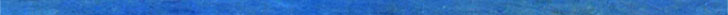# Geometry Problem 1284. Two Equilateral Triangle, Midpoint, Congruence. School, College. Euclidean Geometry.The figure below shows two equilateral triangles ABC and CDE. If F, G, and H are the midpoints of AC, BD, and CE, respectively, prove that the triangle FGH is equilateral.